Measures of Dispersion on Grouped Data
(also see Measures of Dispersion)

Problem:
 Data Entry Frequency 100 8 150 15 200 21 250 14 300 5

For the data set shown in this table, find the range, the standard deviation, and the variance to the nearest hundredth.

Since this question deals with the complete set, we will be using "population" form, not sample form.

For central tendency on grouped data, see Mean, Mode, Median with Grouped Data.

Solution:
To find the range:  No need for calculator work for the range.  It is easily observed from the table.
Range = 300 - 100 = 200.

To find standard deviation:  Remember, we are looking for "population" form which will be found using 1-Var Stats.

Enter the "Data Entry" into L1 and the "Frequency" into L2.  Go to one-variable stats to find "population" standard deviation.
STAT
CALC  #1 1-Var Stats
Be sure to use parameters L1, L2 to indicate both the values AND their frequencies.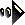NOTE! The standard deviation found in the CATALOG, stdDev, and also found by 2nd LIST → MATH #7 stdDev are both Sample standard deviations.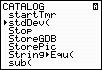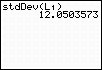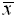= mean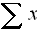= sum of the data= sum of squares of the data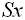= sample standard deviation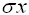= population standard deviation n = sample size (# of pieces of data)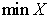= smallest data entry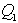= first quartile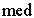= median (second quartile)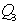= third quartile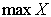= largest data entry

Population Standard Deviation = 56.42

To find variance:   The "population" variance is the square of the population standard deviation.

The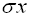symbol is under VARS - #5 Statistics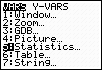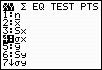NOTE!  The variance found in the CATALOG and also found by 2nd List → MATH #8 variance are both Sample variances.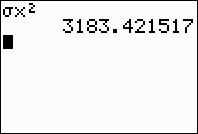Population Variance = 3183.42Finding Your Way Around TABLE of  CONTENTS